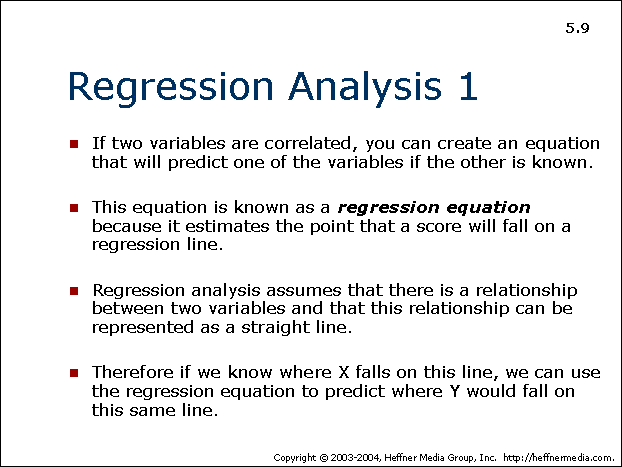advertisement

# 09 – Regression Analysis## Regression Analysis 1

• If two variables are correlated, you can create an equation that will predict one oft he variables if the other is known.
• This equation is known as a regression analysis because it estimates the point that a score will fall on a regression line.
• Regression analysis assumes that there is a relationship between two variables and that this relationship can be represented as a straight line.
• Therefore if we know where X falls on this line, we can use the regression equation to predict where Y could fall on this same line.